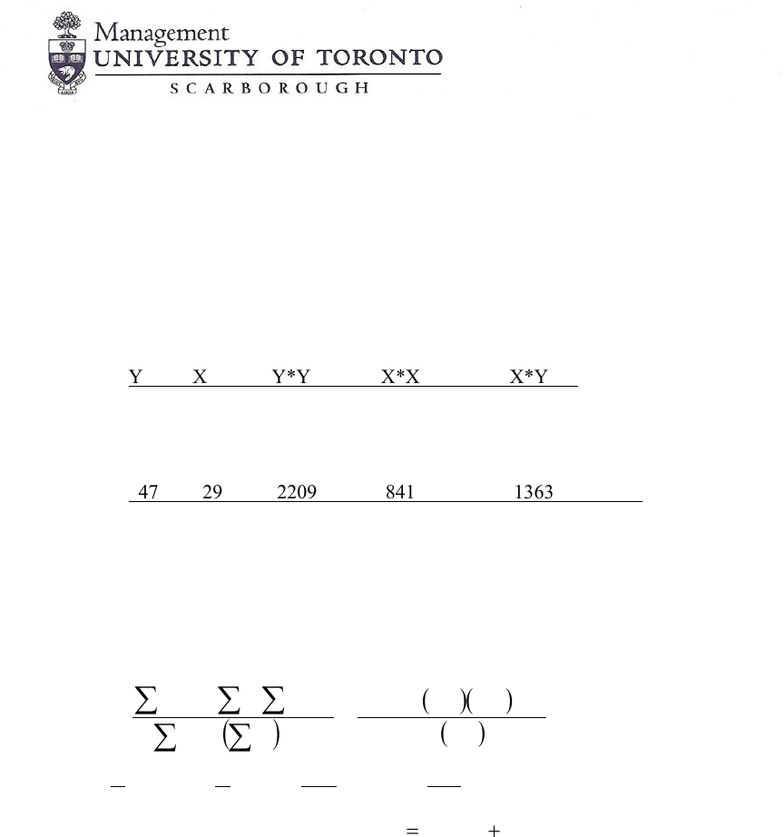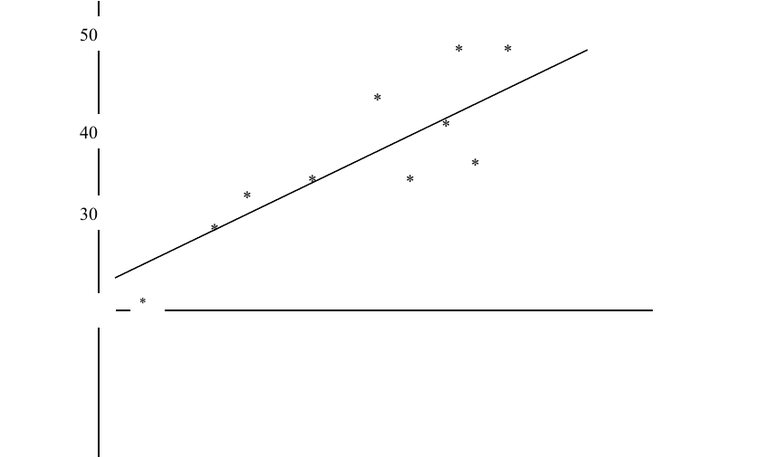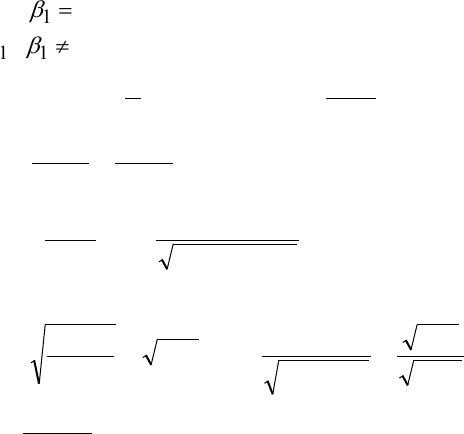# MGEB12H3 Lecture Notes - Lecture 5: Confidence Interval, Multicollinearity, Standard Deviation

26 views8 pages
School
UTSC
Department
Economics for Management Studies
Course
MGEB12H3
ProfessorMGEB12: Quantitative Methods in Economics-II
TUTORIAL-5
Question-1: A company sells chirping crickets (a type of Bug), found only in remote bush
locations. The company claims that the number of seconds between chirps of the bug (Y,
measured in seconds) is determined by the temperature (X, measured in C0). An experiment was
conducted on a bug at various temperatures and the time between chirps measured. The data
obtained and partially summarized in the following table:
Y X Y*Y X*X X*Y
21 -10 441 100 -210
28 -4 784 16 -112
. . . . .
. . . . .
47 29 2209 841 1363
Total 364 130 13862 3296 5603
[Note the sample size is 10]
a) Find the estimated simple regression equation and interpret the coefficients. (One sentence for
each coefficient)
Solution:
( )
( )( )
( )
5423.0
10/1303296
10/3641305603
/
/
22
2
1=
=
=
nxx
nyxyx
b
ii
iiii
350.29
10
130
5423.0
10
36411
10
=
==
ii
x
n
by
n
b
The estimated regression equation is
)(5423.0350.29 XY +=
.
Interpretation: One C0 increase in the temperature is associated with an increase of
0.5423 seconds between chirps of the bug.
Intercept: When the temperature is zero the average number of seconds between
chirps of the bug is on average 29.350.
Unlock document

This preview shows pages 1-3 of the document.
Unlock all 8 pages and 3 million more documents.Page 2 of 8
b) Add the fitted regression line to the plot. Which assumptions of the linear regression model
appear to be violated? Explain in one sentence why?
The assumption of Homocedasticity appears to be violated: variance increases with
temperature.
Time
Temperature
-10
0
10
20
30
30
40
50
*
*
*
*
*
*
*
*
*
Unlock document

This preview shows pages 1-3 of the document.
Unlock all 8 pages and 3 million more documents.Page 3 of 8
c) Using your regression can you infer that the number of seconds between chirps of the
bug is determined by the temperature? To answer this question you need to do a test and
Solution: Using the regression we can only infer whether there is an association or not.
The causality requested is by-product of the sample design. Since the sample
appears experimental if we establish a significant association. (Note if the sample
was observational this conclusion would not have been possible) It will be
sufficient to infer that the number of seconds between chirps of the bug is
determined by the temperature
0:
10
=
β
H
(i.e., Model is not significant)
0: 11
β
H
(i.e., Model is significant)
( )
195.5
1044.0
5423.0
1044.0
1606
5.17
)1(
,5.17
8
02.140
02.1404.612)7714.01(
7714.08783.0
4.6121606
871
9
4.612
110
4.612
4.612
10
364
13862
1
3
2
2
2
2
2
2
2
2
2
1
==
=
===
=×==
==
×
=
=
=
=
=== ∑ ∑
t
Sn
S
SS
SS
S
R
S
y
n
ySST
x
b
yx
xy
y
ii
ε
ε
p-value is very small (<0.01) therefore the regression is significant. You can also use t
from the table = 2.306 => significant at 5%.
Reject the null => the regression is significant.
Unlock document

This preview shows pages 1-3 of the document.
Unlock all 8 pages and 3 million more documents.